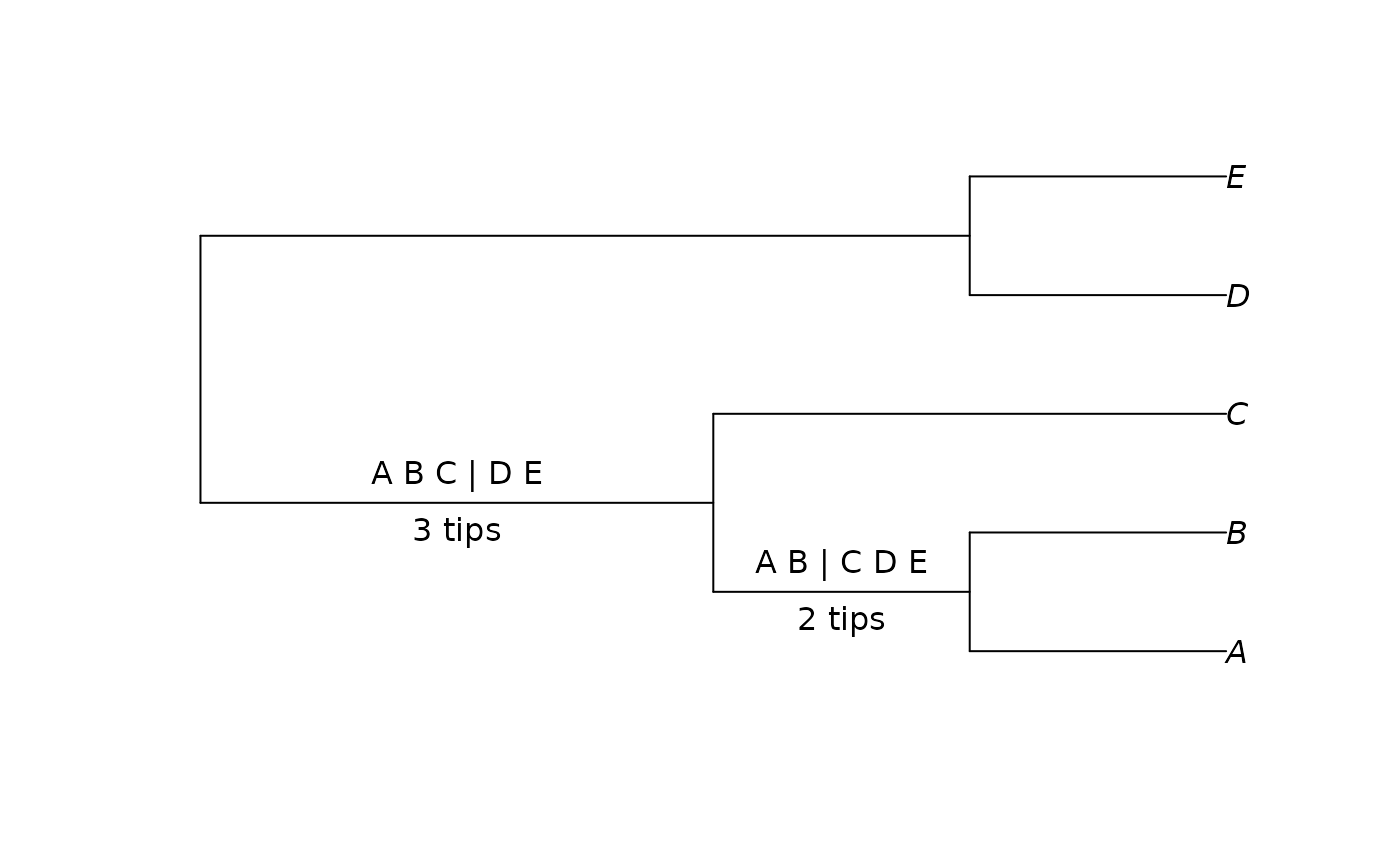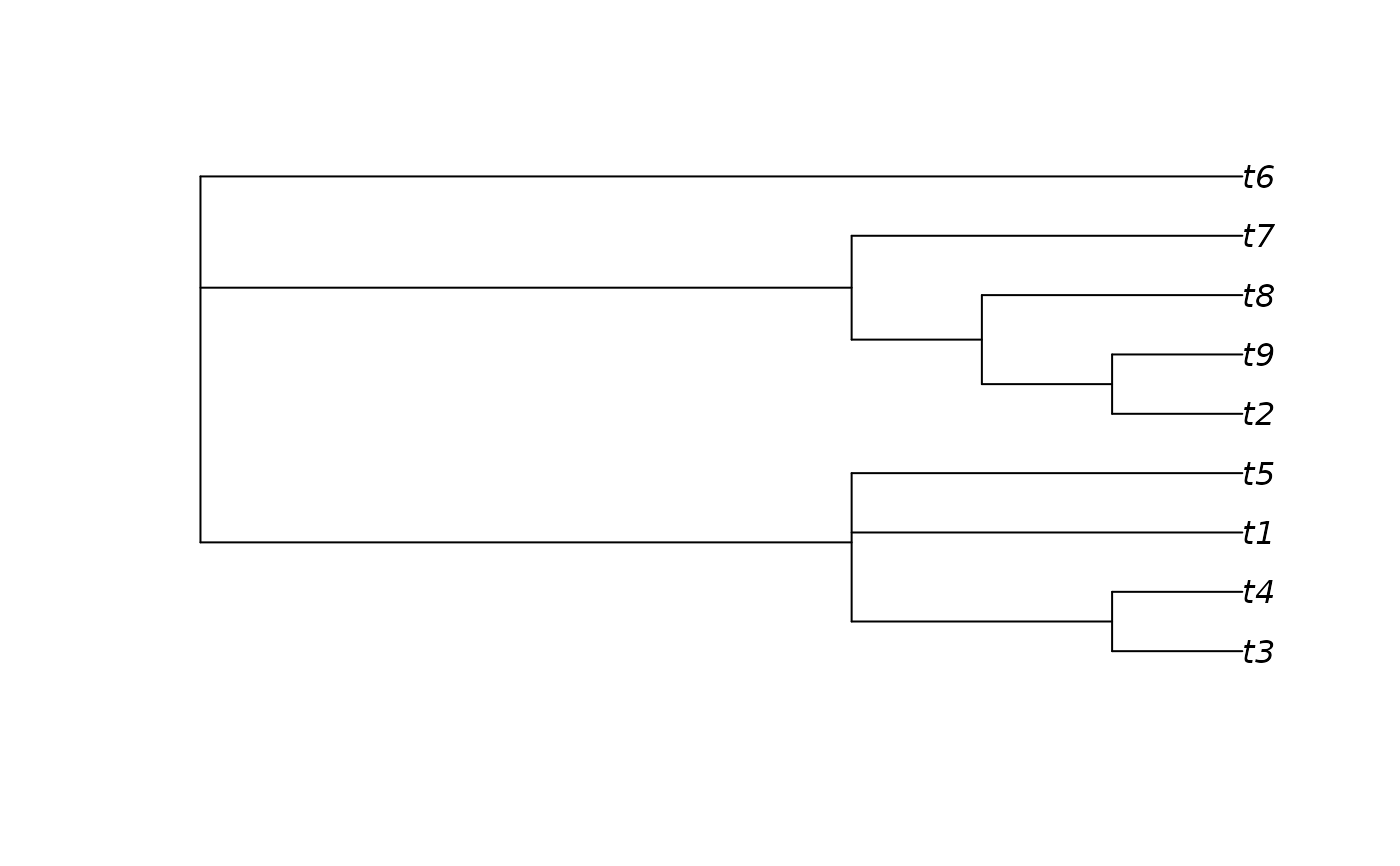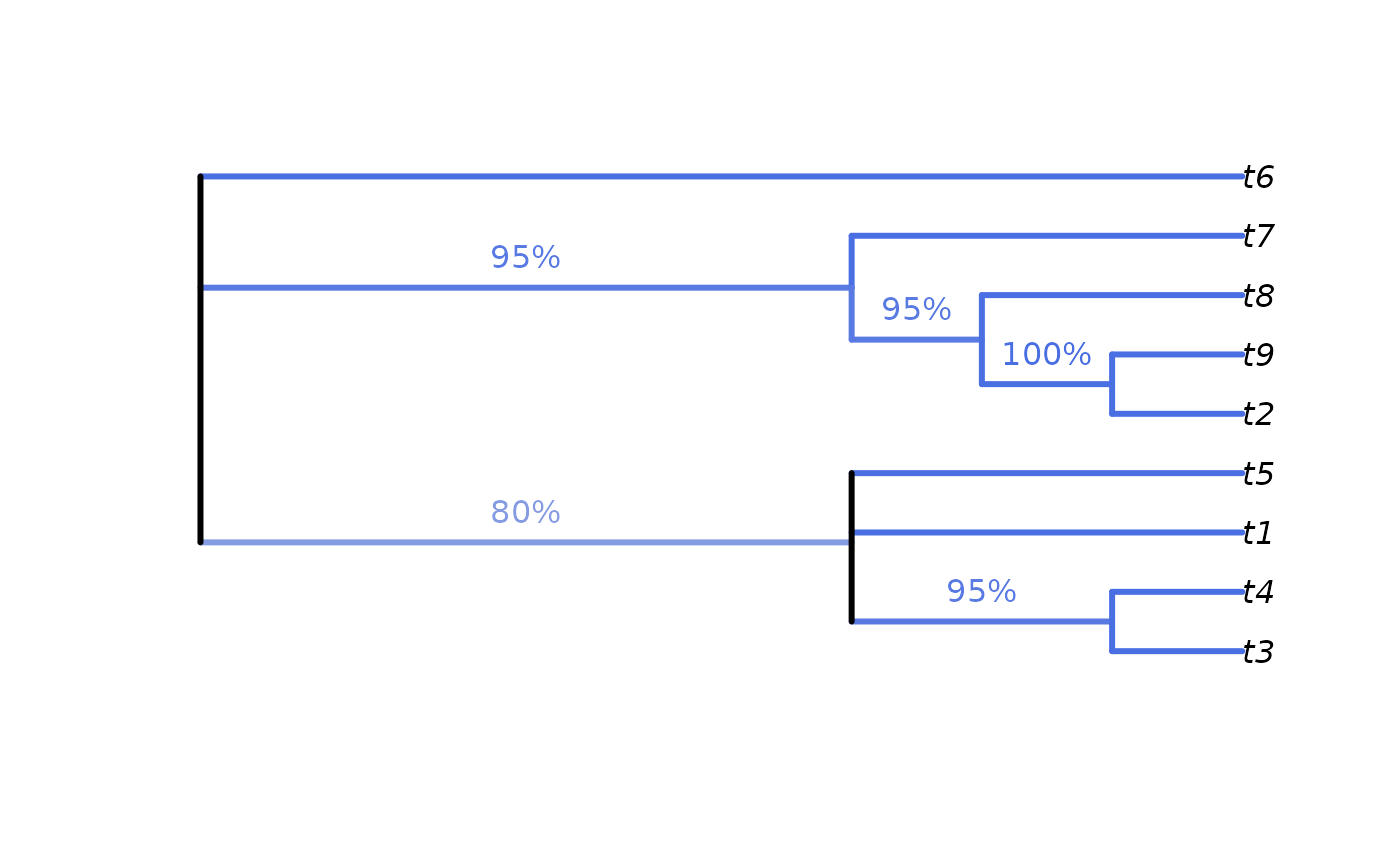Labels the edges associated with each split on a plotted tree.

LabelSplits(tree, labels = NULL, unit = "", ...)

## Arguments

tree

A tree of class phylo.

labels

Named vector listing annotations for each split. Names should correspond to the node associated with each split; see as.Splits() for details. If NULL, each splits will be labelled with its associated node.

unit

Character specifying units of labels, if desired. Include a leading space if necessary.

...

Additional parameters to ape::edgelabels().

## Value

LabelSplits() returns invisible(), after plotting labels on each relevant edge of a plot (which should already have been produced using plot(tree)).

## Details

As the two root edges of a rooted tree denote the same split, only the rightmost (plotted at the bottom, by default) edge will be labelled. If the position of the root is significant, add a tip at the root using AddTip().

Calculate split support: SplitFrequency()

Colour labels according to value: SupportColour()

Other Splits operations: NSplits(), NTip(), PolarizeSplits(), SplitFrequency(), SplitsInBinaryTree(), Splits, TipLabels(), TipsInSplits(), match.Splits, xor()

## Examples

tree <- BalancedTree(LETTERS[1:5])
splits <- as.Splits(tree)
plot(tree)
LabelSplits(tree, as.character(splits), frame = "none", pos = 3L)
LabelSplits(tree, TipsInSplits(splits), unit = " tips", frame = "none",
pos = 1L)# An example forest of 100 trees, some identical
forest <- as.phylo(c(1, rep(10, 79), rep(100, 15), rep(1000, 5)), nTip = 9)

# Generate an 80% consensus tree
cons <- ape::consensus(forest, p = 0.8)
plot(cons)# Calculate split frequencies
splitFreqs <- SplitFrequency(cons, forest)

# Optionally, colour edges by corresponding frequency.
# Note that not all edges are associated with a unique split
# (and two root edges may be associated with one split - not handled here)
edgeSupport <- rep(1, nrow(cons$edge)) # Initialize trivial splits to 1 childNode <- cons$edge[, 2]
edgeSupport[match(names(splitFreqs), childNode)] <- splitFreqs / 100

plot(cons, edge.col = SupportColour(edgeSupport), edge.width = 3)

# Annotate nodes by frequency
LabelSplits(cons, splitFreqs, unit = "%",
col = SupportColor(splitFreqs / 100),
frame = "none", pos = 3L)